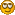###Recent Posts

Pages: 1 2 3 4 5 6  8 9 10
61
##### Development / Re: C::B dark mode in Windows
« Last post by omlk on November 23, 2023, 08:59:55 pm »
Hello,
I am trying to enable dark mode in MSW build. I think this may be a cool feature for some people who tend to use dark mode in everything.
This is easy if I compile it with wxWidgets 3.3 (from GitHub), one line of code is needed after app starts (https://docs.wxwidgets.org/latest/classwx_app.html#af8c93d7e3345e62a58325f3ab1d158d6)

I will post the code soon. However, I cannot find where in the code of C::B are the default values for editor colors. Where in the source code can I find the colors for editor background/foreground and syntax highlighting? Background is white by default even when using the dark mode.
I hope it's not photoshopped. I'm joking. You are still the first to do it (congratulations), but can you use any color in the color palette instead of the black interface, for example?
62
##### Development / Re: RFC about pane translation
« Last post by omlk on November 23, 2023, 08:49:16 pm »
Are you talking about these pieces of XML in the default.conf file?it is possible to move these language-related pieces to the lang/en subfolder; lang/fr; lang/it.
Code
``default.conflang/  it.xml  en.xml``
Can we import an XML file into another XML file?

Code
``<DATA>                        <str>                            <![CDATA[layout2|name=ManagementPane;caption=Management;state=2099198;dir=4;layer=1;row=0;pos=0;prop=100000;bestw=200;besth=846;minw=100;minh=100;maxw=-1;maxh=-1;floatx=-1;floaty=-1;floatw=-1;floath=-1|name=MessagesPane;caption=Logs & others;state=2099198;dir=3;layer=0;row=0;pos=0;prop=100000;bestw=1164;besth=150;minw=-1;minh=-1;maxw=-1;maxh=-1;floatx=-1;floaty=-1;floatw=-1;floath=-1|name=CallStackPane;caption=Call stack;state=2099199;dir=4;layer=0;row=0;pos=0;prop=100000;bestw=150;besth=150;minw=150;minh=150;maxw=-1;maxh=-1;floatx=200;floaty=150;floatw=450;floath=150|name=BreakpointsPane;caption=Breakpoints;state=2099199;dir=4;layer=0;row=0;pos=0;prop=100000;bestw=350;besth=250;minw=150;minh=150;maxw=-1;maxh=-1;floatx=200;floaty=150;floatw=350;floath=250|name=CPURegistersPane;caption=CPU Registers;state=2099199;dir=4;layer=0;row=0;pos=0;prop=100000;bestw=350;besth=250;minw=150;minh=150;maxw=-1;maxh=-1;floatx=200;floaty=150;floatw=350;floath=250|name=DisassemblyPane;caption=Disassembly;state=2099199;dir=4;layer=0;row=0;pos=0;prop=100000;bestw=350;besth=250;minw=150;minh=150;maxw=-1;maxh=-1;floatx=200;floaty=150;floatw=350;floath=250|name=ExamineMemoryPane;caption=Memory;state=2099199;dir=4;layer=0;row=0;pos=0;prop=100000;bestw=450;besth=250;minw=350;minh=150;maxw=-1;maxh=-1;floatx=200;floaty=150;floatw=450;floath=250|name=ThreadsPane;caption=Running threads;state=2099199;dir=4;layer=0;row=0;pos=0;prop=100000;bestw=350;besth=75;minw=250;minh=75;maxw=-1;maxh=-1;floatx=200;floaty=150;floatw=450;floath=75|name=WatchesPane;caption=Watches;state=2099199;dir=4;layer=0;row=0;pos=0;prop=100000;bestw=150;besth=250;minw=150;minh=150;maxw=-1;maxh=-1;floatx=200;floaty=150;floatw=150;floath=250|name=MainPane;caption=;state=768;dir=5;layer=0;row=0;pos=0;prop=100000;bestw=20;besth=20;minw=-1;minh=-1;maxw=-1;maxh=-1;floatx=-1;floaty=-1;floatw=-1;floath=-1|name=ScriptConsole;caption=Scripting console;state=2099199;dir=4;layer=0;row=0;pos=0;prop=100000;bestw=253;besth=100;minw=100;minh=100;maxw=-1;maxh=-1;floatx=300;floaty=200;floatw=-1;floath=-1|name=JumpTrackerPane;caption=JumpTracker View;state=2099198;dir=4;layer=1;row=0;pos=0;prop=100000;bestw=300;besth=300;minw=30;minh=40;maxw=-1;maxh=-1;floatx=200;floaty=150;floatw=300;floath=400|name=CodeSnippetsPane;caption=CodeSnippets;state=2099199;dir=4;layer=1;row=0;pos=0;prop=100000;bestw=300;besth=400;minw=30;minh=40;maxw=-1;maxh=-1;floatx=200;floaty=150;floatw=300;floath=400|name=DefMimeHandler_HTMLViewer;caption=HTML viewer;state=2099199;dir=4;layer=0;row=0;pos=0;prop=100000;bestw=350;besth=250;minw=150;minh=150;maxw=-1;maxh=-1;floatx=200;floaty=150;floatw=350;floath=250|name=FCallTree;caption=Fortran Call/Called-By tree;state=2099199;dir=4;layer=0;row=0;pos=0;prop=100000;bestw=200;besth=250;minw=150;minh=150;maxw=-1;maxh=-1;floatx=200;floaty=150;floatw=200;floath=250|name=MANViewer;caption=Man/Html pages viewer;state=2099198;dir=2;layer=0;row=0;pos=0;prop=100000;bestw=320;besth=240;minw=240;minh=160;maxw=-1;maxh=-1;floatx=200;floaty=150;floatw=320;floath=240|name=HighlightedOccurrences;caption=Highlighted Occurrences;state=2099198;dir=4;layer=1;row=0;pos=0;prop=100000;bestw=150;besth=100;minw=50;minh=50;maxw=-1;maxh=-1;floatx=200;floaty=150;floatw=100;floath=150|name=OpenFilesPane;caption=Open files list;state=2099198;dir=4;layer=1;row=0;pos=0;prop=100000;bestw=150;besth=100;minw=50;minh=50;maxw=-1;maxh=-1;floatx=200;floaty=150;floatw=100;floath=150|name=TodoListPanev2.0.0;caption=Todo list;state=2099199;dir=4;layer=0;row=0;pos=0;prop=100000;bestw=352;besth=94;minw=252;minh=94;maxw=-1;maxh=-1;floatx=200;floaty=150;floatw=352;floath=94|name=Tools;caption=Tool Output;state=2099199;dir=4;layer=0;row=0;pos=0;prop=100000;bestw=400;besth=300;minw=200;minh=150;maxw=-1;maxh=-1;floatx=200;floaty=150;floatw=400;floath=300|name=MainToolbar;caption=Main Toolbar;state=2108158;dir=1;layer=10;row=0;pos=0;prop=100000;bestw=365;besth=32;minw=-1;minh=-1;maxw=-1;maxh=-1;floatx=-1;floaty=-1;floatw=-1;floath=-1|name=CompilerToolbar;caption=Compiler Toolbar;state=2108158;dir=1;layer=10;row=0;pos=365;prop=100000;bestw=325;besth=32;minw=-1;minh=-1;maxw=-1;maxh=-1;floatx=-1;floaty=-1;floatw=-1;floath=-1|name=DebuggerToolbar;caption=Debugger Toolbar;state=2108158;dir=1;layer=10;row=0;pos=690;prop=100000;bestw=349;besth=32;minw=-1;minh=-1;maxw=-1;maxh=-1;floatx=-1;floaty=-1;floatw=-1;floath=-1|name=CodeCompletionToolbar;caption=Code completion Toolbar;state=2108158;dir=1;layer=10;row=1;pos=0;prop=100000;bestw=946;besth=32;minw=-1;minh=-1;maxw=-1;maxh=-1;floatx=-1;floaty=-1;floatw=-1;floath=-1|name=BrowseTrackerToolbar;caption=BrowseTracker Toolbar;state=2108158;dir=1;layer=10;row=1;pos=946;prop=100000;bestw=194;besth=32;minw=-1;minh=-1;maxw=-1;maxh=-1;floatx=-1;floaty=-1;floatw=-1;floath=-1|name=DoxyBlocksToolbar;caption=DoxyBlocks Toolbar;state=2108158;dir=1;layer=10;row=2;pos=0;prop=100000;bestw=241;besth=32;minw=-1;minh=-1;maxw=-1;maxh=-1;floatx=-1;floaty=-1;floatw=-1;floath=-1|name=FortranProjectToolbar;caption=FortranProject Toolbar;state=2108158;dir=1;layer=10;row=2;pos=241;prop=100000;bestw=93;besth=32;minw=-1;minh=-1;maxw=-1;maxh=-1;floatx=-1;floaty=-1;floatw=-1;floath=-1|name=IncrementalSearchToolbar;caption=Incremental search Toolbar;state=2108158;dir=1;layer=10;row=2;pos=334;prop=100000;bestw=388;besth=32;minw=-1;minh=-1;maxw=-1;maxh=-1;floatx=-1;floaty=-1;floatw=-1;floath=-1|name=NassiShneidermanPluginToolbar;caption=Nassi-Shneiderman diagram Toolbar;state=2108158;dir=1;layer=10;row=3;pos=0;prop=100000;bestw=513;besth=32;minw=-1;minh=-1;maxw=-1;maxh=-1;floatx=-1;floaty=-1;floatw=-1;floath=-1|name=ThreadSearchToolbar;caption=Thread search Toolbar;state=2108158;dir=1;layer=10;row=3;pos=513;prop=100000;bestw=265;besth=32;minw=-1;minh=-1;maxw=-1;maxh=-1;floatx=-1;floaty=-1;floatw=-1;floath=-1|]]>                        </str>                    </DATA>``
63
##### Development / Re: RFC about pane translation
« Last post by Pecan on November 23, 2023, 08:26:56 pm »
Will keeping different personalities for each language work for you?
That, at least, will write a separate .conf file for each language.
64
##### Development / Re: RFC about pane translation
« Last post by gd_on on November 23, 2023, 08:07:56 pm »
As you know, these strings are saved in a .conf file, default.conf for me (in items caption=something).
Do you know why has been made this choice ? other strings are translated on the fly, but why not these ones ?
Apparently, they are recreated at the next C::B start, if you delete the default.conf, but this is not a good solution because you loose all other config adjustements !
May be you can recreate those strings, and only them, keeping all other settings, when you change the interface language.
65
##### Development / RFC about pane translation
« Last post by Miguel Gimenez on November 23, 2023, 07:20:13 pm »
Translating the pane labels as I did in r13179 works, but has a nasty side effect: When saving the perspective the translated labels are saved, overwriting the original english labels that get lost forever.

In brief, the first time you select a non-english language the labels will get stuck in said language and you will not be able to see them in other language.

I am thinking about reverting r13179, this would leave the AUI panes' labels permanently in english, but at least they will not be in russian as I have them currently with all other texts in spanish.

Ideas? Opinions?
66
##### General (but related to Code::Blocks) / Re: Welcome Newcomers - PLEASE READ!!!
« Last post by Mattia2341 on November 23, 2023, 06:57:46 pm »
#include <iostream>
using namespace std;
int main(); {
cout<<"hi, i'm human\n";
return 0;
}
67
##### Development / Re: C::B dark mode in Windows
« Last post by Miguel Gimenez on November 23, 2023, 04:30:09 pm »
Code
``wxColor fg = LoadFromLexer(FOREGROUND);wxColor bg = LoadFromLexer(BACKGROUND);if (DarkMode){    fg.SetRGBA(fg.GetRGBA() ^ 0x00FFFFFF);    bg.SetRGBA(bg.GetRGBA() ^ 0x00FFFFFF);}``
inverting them again when saving.
68
##### Development / Re: C::B dark mode in Windows
« Last post by cacb on November 23, 2023, 03:43:03 pm »
Dark mode in Code::Blocks is a good idea, great work!

If that can be made a standard and easy to use on/off option in Code::Blocks I would probably use it. It also keeps Code::Blocks up to date with similar features in other IDEs.
69
##### Development / Re: C::B dark mode in Windows
« Last post by ollydbg on November 23, 2023, 02:45:45 pm »
The screen shot shown in the first post looks nice! good work!
70
##### General (but related to Code::Blocks) / Re: Creating Fortran Project
« Last post by Miguel Gimenez on November 23, 2023, 12:07:24 pm »
The folder must be a writeable one; do not use c:\, a system folder or C::B installation folder. A folder under "My documents" is usually fine. Do not use spaces in the folder name.

The include files can be wherever you want, just add them to the compiler search directorioes.

The binary will be stored in the folder you specify in the properties of the project, you can write your output files in that folder. Remeber to set the execution folder to the bin one or your files will be written in the source folder.
Pages: 1 2 3 4 5 6  8 9 10